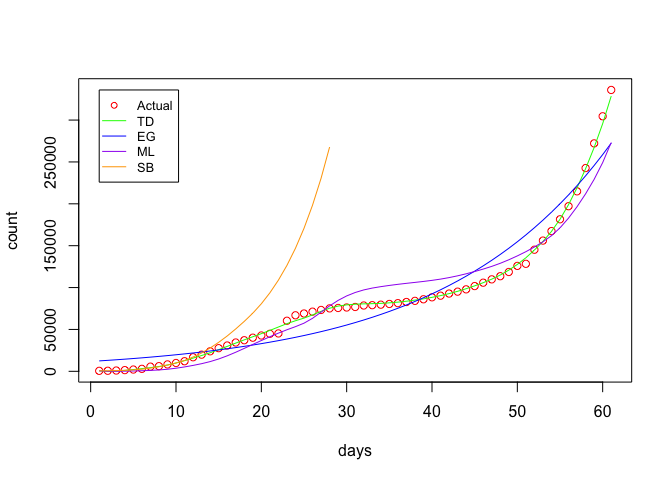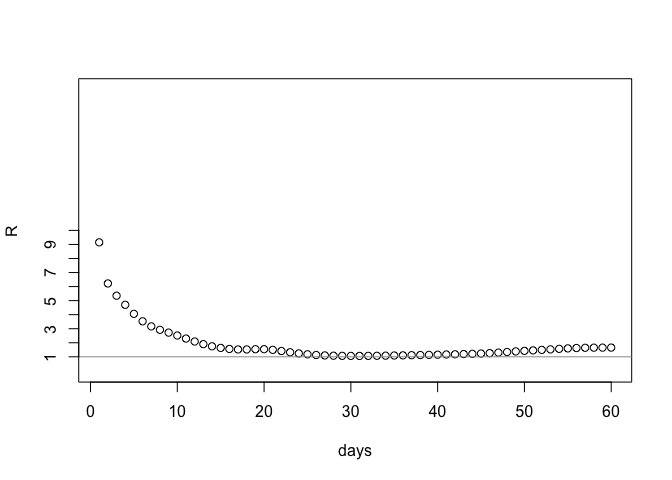Here I take the R0 package to estimate the reproduction number for COVID-19. NOTE: my aim is to investigate the R0 package rather than provide sound predictions of COVID-19’s future behaviour. In particular I have guessed the generation time.

Load the data from the 2019 Novel Coronavirus COVID-19 (2019-nCoV) Data Repository by Johns Hopkins CSSE:

confirmed <- read.csv(url("https://raw.githubusercontent.com/CSSEGISandData/2019-nCoV/master/csse_covid_19_data/csse_covid_19_time_series/time_series_19-covid-Confirmed.csv"))

This data contains a row per state/province and a column per reported case numbers - on a particular date. Let’s tidy this into count per day number since the start.

confirmed <- subset(confirmed, select = -c(Lat, Long))

sums <- colSums(confirmed[,-match(c("Province.State", "Country.Region"), names(confirmed))], na.rm=TRUE)

sumsByDateCode <- as.data.frame(t(t(sums)))
colnames(sumsByDateCode) <- c("count")

sumsByDateCode$datecode <- rownames(sumsByDateCode) dailyCounts <- mutate(sumsByDateCode, date = mdy(substring(datecode,2))) dailyCounts$day <- seq.int(nrow(dailyCounts))

Now use the R0 package to estimate the reproduction number. I need a generation time, which we don’t have to hand, so I take the values use for SARS - a mean of 5, standard deviation 1.9 - as described here. The different estimation methods (time dependent, exponential growth etc.) are described there too.

mgt <- generation.time("gamma", c(5, 1.9))

est <- estimate.R(dailyCounts\$count, methods=c("TD", "EG", "ML", "SB"), GT=mgt)

We can plot the actual and predicted values using the different estimation methods:The time-dependent method seems to fit the best. Here are the RMSE values for the different methods:

TD EG ML SB
2809.946 19230.84 18054.14 NA

Here is the range of the reproduction number thus estimated using the “time dependendent” method:

##    Min. 1st Qu.  Median    Mean 3rd Qu.    Max.
##   1.062   1.160   1.474   1.889   1.654   9.150

Finally, here is a plot of estimated reproduction number (using the time-dependent method) over time:The reproduction number has been reduced considerably over the last month or so, but is still above 1.